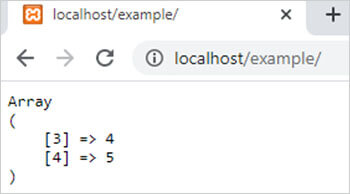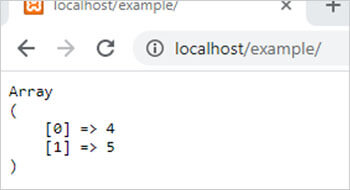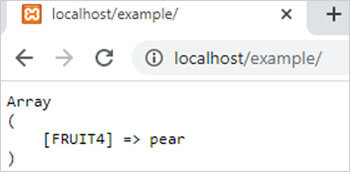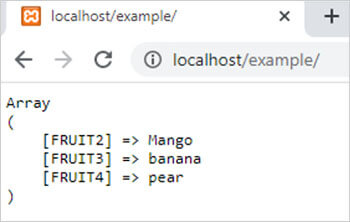array_slice() function in php || PHP Array Slice Function# array_slice() function in php

2139

array_slice() function of PHP is used to retrieve the part of the array by applying some conditions. It is an inbuilt function of PHP.

Syntax:

array_slice(\$array,\$starting_index, \$length, preserve);

here,

• \$array is a PHP array. It is mandatory.
• \$starting_index is the starting index from where the function starts to slice the array. It is a numeric value. It is mandatory.
• \$length is also a numeric value that specifies the length of the newly created array. It is also mandatory.
• preserve is a Boolean value which can be either TRUE or FALSE. It is optional. It preserves the order of keys at the value TRUE and reset or re-indexed the keys if the value is FALSE.

Example 1:

```<?php
\$arr1=array(1,2,3,4,5,6,7,8);
\$result=array_slice(\$arr1,3,2,TRUE); // preserve value TRUE
echo "<pre>";
print_r(\$result);
?>```

Output:Example 2:

```<?php
\$arr1=array(1,2,3,4,5,6,7,8);
\$result=array_slice(\$arr1,3,2,FALSE); // preserve value FALSE re indexed array from 0
echo "<pre>";
print_r(\$result);
?>```

Output:Example 3:

array_slice() with associative array. Preserve does not reflect the keys in the case of an associative array.

```<?php
\$arr1=array("FRUIT1"=>"apple","FRUIT2"=>"Mango","FRUIT3"=>"banana","FRUIT4"=>"pear");
\$result=array_slice(\$arr1,3,2,TRUE);
echo "<pre>";
print_r(\$result);
?>```

Output:Example 4:

```<?php
\$arr1=array("FRUIT1"=>"apple","FRUIT2"=>"Mango","FRUIT3"=>"banana","FRUIT4"=>"pear");
\$result=array_slice(\$arr1,1,3,FALSE);
echo "<pre>";
print_r(\$result);
?>```

Output:Share:## Big Hosting Days

Upto 75@ OFF on Web Hosting

## Get a .COM for just \$6.98

Secure Domain for a Mini Price